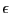Next: Analyzing trigonometric equations Up: Inflation Previous: Mathematical background   Contents

Implementation

int ALIAS_Epsilon_Inflation(int Dimension,int Dimension_Eq,
INTERVAL_VECTOR (* TheIntervalFunction)(int,int,INTERVAL_VECTOR &),
INTERVAL_MATRIX (* Gradient)(int, int, INTERVAL_VECTOR &),
INTERVAL_MATRIX (* Hessian)(int, int, INTERVAL_VECTOR &),
VECTOR &X0,
INTERVAL_VECTOR &B)
Note that the Hessian argument is not used in this procedure. This routine will return 1 if the inflation has succeeded. The value ofcan be found in the global variable. ALIAS_Eps_Inflation

Jean-Pierre Merlet 2012-12-20